Email Us: info@lupinepublishers.comCall Us: +1 (914) 407-6109   57 West 57th Street, 3rd floor, New York - NY 10019, USA

# Lupine Publishers Group

## Lupine Publishers

Submit Manuscript

ISSN: 2637-6652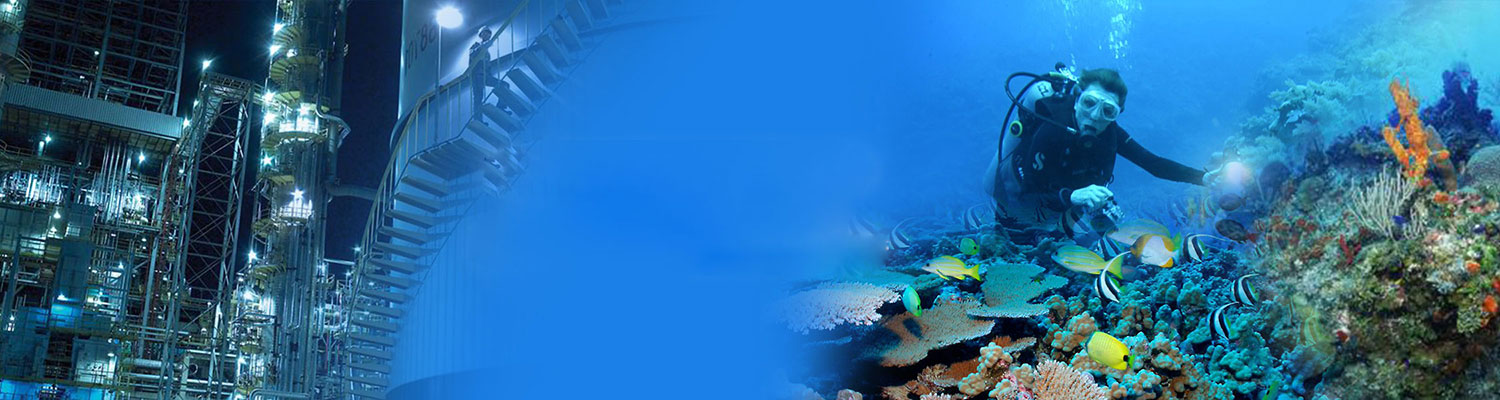# Seismo Mechanical Force Fractal Dimension for Characterizing Shajara Reservoirs of the Permo- Carboniferous Shajara Formation, Saudi Arabia Volume 2 - Issue 2

Khalid Elyas Mohamed Elameen Alkhidir*

• Department of Petroleum and Natural Gas Engineering, King Saud University, Saudi Arabia

Received: September 09, 2018;   Published: September 21, 2018

Corresponding author: Khalid Elyas Mohamed Elameen Alkhidir, Department of Petroleum and Natural Gas Engineering, college of Engineering, King Saud University, Riyadh, Saudi Arabia

## Abstract

The quality and assessment of a reservoir can be recognized in details by the presentation of seismo mechanical force. This research targets to calculate fractal dimension from the relationship among seismo mechanical force, maximum seismo mechanical force and wetting phase saturation and to approve it by the fractal dimension derived from the relationship among capillary pressure and wetting phase saturation. In this research, porosity was measured on real collected sandstone samples and permeability was calculated theoretically from capillary pressure profile measured by mercury intrusion contaminating the pores of sandstone samples in consideration. Two equations for calculating the fractal dimensions have been employed. The first one defines the functional relationship between wetting phase saturation, seismo mechanical force, maximum seismo mechanical force and fractal dimension. The second equation involves to the wetting phase saturation as a function of capillary pressure and the fractal dimension. Two procedures for obtaining the fractal dimension have been utilized. The first procedure was done by plotting the logarithm of the ratio between seismo mechanical force and maximum seismo mechanical force versus logarithm wetting phase saturation. The slope of the first procedure = 3-Df (fractal dimension). The second procedure for obtaining the fractal dimension was determined by plotting the logarithm of capillary pressure versus the logarithm of wetting phase saturation. The slope of the second procedure = Df-3. On the basis of the obtained results of the fabricated stratigraphic column and the attained values of the fractal dimension, the sandstones of the Shajara reservoirs of the Shajara Formation were divided here into three units. The gained units from bottom to top are: Lower Shajara seismo mechanical force Fractal Dimension Unit, Middle Shajara seismo mechanical force Fractal dimension Unit, and Upper Shajara seismo mechanical force Fractal Dimension Unit. The results show similarity between seismo mechanical force fractal dimension and capillary pressure fractal dimension. It was also noted that samples with wide range of pore radius were characterized by high values of fractal dimensions due to an increase in their connectivities. In our case, and as conclusions the higher the fractal dimension, the higher the heterogeneity, the higher the permeability, the better the reservoir characteristics.

## Introduction

The wetting phase saturation can be described as function of capillary pressure and fractal dimension was demonstrated by Toledo GT . The Purcell model was found to be the best fit to the experimental data of the wetting phase relative permeability for the cases as long as the measured capillary pressure curve had the same residual saturation as the relative permeability curve was described by Li K and Horne RN . A theoretical model to correlate capillary pressure and resistivity index based on the fractal scaling theory was reported by Li K and Willams W . The fractal dimension resulting from longer transverse NMR relaxation times and lower capillary pressure reflects the volume dimension of larger pores was described by Zhang Z and Weller A . The fractal dimension derived from the short NMR relaxation times is similar to the fractal dimension of the internal surface was described by Zhang Z and Weller A . The fractal dimensions can be used to represent the complexity degree and heterogeneity of pore structure, and the coexistence of dissolution pores and large intergranular pores of Donghetang sandstones contributes to a heterogeneous pore throat distribution and a high value of fractal dimension was reported by Wang Z . The relationship among capillary pressure (PC), nuclear magnetic transverse relaxation time (T2) and resistivity index (I) was studied by Guo Yh . An increase of bubble pressure fractal dimension and pressure head fractal dimension and decreasing pore size distribution index and fitting parameters m * n due to possibility of having interconnected channels was confirmed by Alkhidir KEME . An increase of fractal dimension with increasing arithmetic, geometric relaxation time of induced polarization, permeability and grain size was investigated by Alkhidir KEME [8-10]. An increase of seismo electric field and resistivity fractal dimensions with increasing permeability and grain size was described by Alkhidir KEME [11-13]. An increase of electric potential gradient and electro kinetic fractal dimensions with increasing permeability and grain size was reported by Alkhidir KEME [14,15]. An increase of electric potential energy fractal with increasing permeability and grain size was defined by Alkhidir KEME .

## Method and Materials

Samples were collected from the surface type section of the Shajara reservoirs of the Permo-Carboniferous Shajara formation at latitude 26° 52′ 17.4″, longitude 43° 36′ 18″. Porosity was measured, and permeability was derived from the measured capillary pressure data.

The seismo mechanical force fractal dimension can be scaled as

Where Sw, the water saturation, F the seismo mechanical force in Newton, Fmax, the maximum seismo mechanical force in Newton and Df the fractal dimension.

Equation 1 can be proofed from electric current density taking into account the electro kinetic effect, angular frequency, seismic displacement and fluid density.

Where J the electric current density in ampere / square meter, CEK the electro kinetic coefficient in ampere /(pascal * meter), ω the seismic angular frequency in hertz, Us the seismic displacement in meter, and ρf the fluid density in kilogram / cubic meter.

Where ρf the density in kilogram / cubic meter, m the mass in kilogram, and V the volume in cubic meter.

Insert equation 3 into equation 2

The mass m can be scaled as

Where F the seismo mechanical force in Newton, g the acceleration in meter/(square second).

Insert equation 5 into equation 4

The volume in equation 6 can be scaled as

Where r the pore radius in meter Insert equation 7 into equation 6

The pore radius of equation 8 after rearrangement will become

The maximum pore radius rmax can be scaled as

Divide equation 9 by equation 10

Equation 11 after simplification will become

Take the third root of equation 12

Equation 13 can also be written as

Take the logarithm of equation 14

Insert equation 16 into equation 15

Equation 17 after log removal will become

Equation 18 the proof of equation 1 which relates the Water saturation, the seismo mechanical force, the maximum seismo mechanical force and the fractal dimension.

The capillary pressure can be scaled as

log Sw = (Df − 3)∗log Pc + cons tan t (19)

Where Sw the water saturation, Pc the capillary pressure and Df the fractal dimension

## Results and Discussion

Based on field observation the Shajara Reservoirs of the Permo- Carboniferous Shajara Formation were divided into three units as described in Figure 1. These units from bottom to top are: Lower Shajara Reservoir, Middle Shajara reservoir, and Upper Shajara Reservoir. Their acquired results of the seismo mechanical force fractal dimension and capillary pressure fractal dimension is displayed in Table 1. Based on the attained results it was found that the seismo mechanical force fractal dimension is equal to the capillary pressure fractal dimension. The maximum value of the fractal dimension was found to be 2.7872 assigned to sample SJ13 from the Upper Shajara Reservoir as verified in Table 1. Whereas the minimum value 2.4379 of the fractal dimension was reported from sample SJ3 from the Lower Shajara reservoir as displayed in Table1. The seismo mechanical force fractal dimension and capillary pressure fractal dimension were observed to increase with increasing permeability as proofed in Table 1 owing to the possibility of having interconnected channels.

The Lower Shajara reservoir was represented by six sandstone samples Figure 1, four of which labeled as SJ1, SJ2, SJ3 and SJ4 as confirmed in Table 1 were selected for capillary pressure measurement. Their positive slopes of the first procedure and negative slopes of the second procedure are delineated in Figures 2-5 and Table 1. Their seismo mechanical force fractal dimension and capillary pressure fractal dimension values are proofed in Table 1. As we progress from sample SJ2 to SJ3 a pronounced reduction in permeability due to compaction was reported from 1955 md to 56 md which reflects decrease in seismo mechanical force fractal dimension and capillary pressure fractal dimension from 2.7748 to 2.4379 as identified in Table 1. Again, an increase in grain size and permeability was verified from sample SJ4 whose seismo mechanical force fractal dimension and capillary pressure fractal dimension was found to be 2.6843 as defined in Table 1.

Table 1: Petrophysical model showing the three Shajara Reservoir Units with their corresponding values of seismo mechanical force dimension and capillary pressure fractal dimension.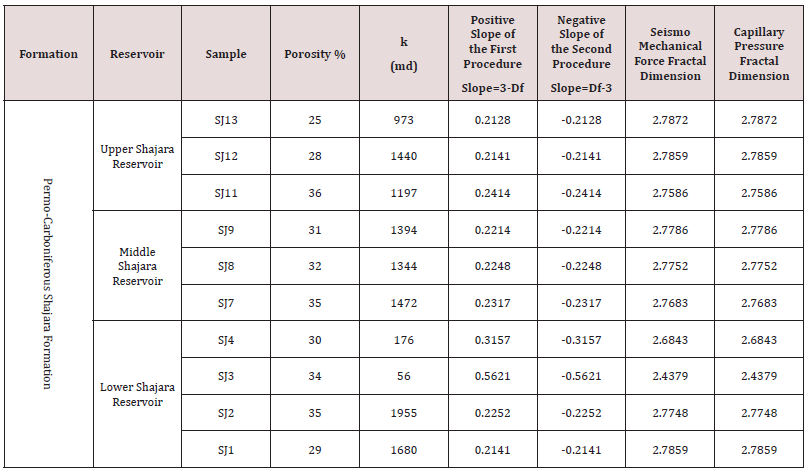Figure 1: Surface type section of the Shajara Reservoirs of the Permo-Carboniferous Shajara Formation, at latitude 26° 52′ 17.4″, longitude 43° 36′ 18″.Figure 2: Log (F1/3/ F1/3max) vs log Sw (blue color) & log Pc vs log Sw (red) color for sample SJ1.Figure 3: Log (F1/3/ F1/3max) vs log Sw (blue color) & log Pc vs log Sw (red) color for sample SJ2.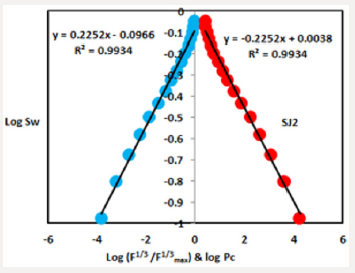Figure 4: Log (F1/3/ F1/3max) vs log Sw (blue color) & log Pc vs log Sw (red) color for sample SJ3.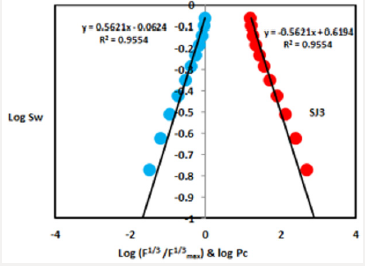Figure 5: Log (F1/3/ F1/3max) vs log Sw (blue color) & log Pc vs log Sw (red) color for sample SJ4.In contrast, the Middle Shajara reservoir which is separated from the Lower Shajara reservoir by an unconformity surface as shown in Figure 1. It was designated by four samples Figure 1, three of which named as SJ7, SJ8, and SJ9 as proved in Table 1 were picked for capillary pressure measurement. Their positive slopes of the first procedure and negative slopes of the second procedure are revealed in Figures 6-8 and Table 1. Their seismo mechanical force fractal dimensions and capillary pressure fractal dimensions show similarities as delineated in Table 1. Their fractal dimensions are higher than those of samples SJ3 and SJ4 from the Lower Shajara Reservoir due to an increase in their permeability as explained in Table 1.

Figure 6: Log (F1/3/ F1/3max) vs log Sw (blue color) & log Pc vs log Sw (red) color for sample SJ7.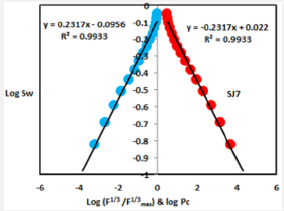Figure 7: Log (F1/3/ F1/3max) vs log Sw (blue color) & log Pc vs log Sw (red) color for sample SJ8.Figure 8: Log (F1/3/ F1/3max) vs log Sw (blue color) & log Pc vs log Sw (red) color for sample SJ8.On the other hand, the Upper Shajara reservoir is separated from the Middle Shajara reservoir by yellow green mudstone as shown in Figure 1. It is defined by three samples so called SJ11, SJ12, SJ13 as explained in Table 1. Their positive slopes of the first procedure and negative slopes of the second procedure are presented in Figures 9-11 and Table 1. Moreover, their seismo mechanical force fractal dimension and capillary pressure fractal dimension is also higher than those of sample SJ3 and SJ4 from the Lower Shajara Reservoir due to an increase in their permeability as clarified in Table 1. ssOverall a plot of seismo mechanical force fractal dimension versus capillary pressure fractal dimension as shown in Figure 12 reveals three permeable zones of varying Petrophysical properties. Such variation in fractal dimension can account for heterogeneity which is a key parameter in reservoir quality assessment. This heterogeneity was also revealed by plotting positive slope of the first procedure versus negative slope of the second procedure as described in Figure 13.

Figure 9: Log (F1/3/ F1/3max) vs log Sw (blue color) & log Pc vs log Sw (red) color for sample SJ1.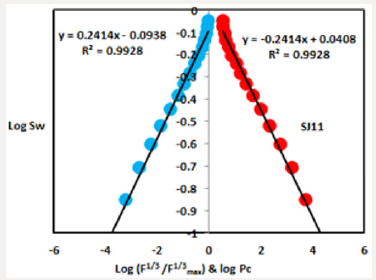Figure 10: Log (F1/3/ F1/3max) vs log Sw (blue color) & log Pc vs log Sw (red) color for sample SJ12.Figure 11: Log (F1/3/ F1/3max) vs log Sw (blue color) & log Pc vs log Sw (red) color for sample SJ13.Figure 12: Seismo mechanical force fractal dimension versus capillary pressure fractal dimension.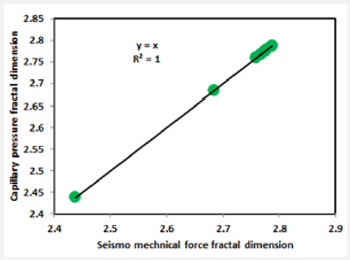Figure 13: Positive slope of the first procedure versus negative slope of the second procedure.## Conclusion

a. The sandstones of the Shajara Reservoirs of the Permo- Carboniferous Shajara formation were divided here into three units based on seismo mechanical force fractal dimension.

b. The gained units from bottom to top are: Lower Shajara Seismo Mechanical Force Fractal Dimension Unit, Middle Shajara Seismo Mechanical Force Fractal Dimension Unit, and Upper Shajara Seismo Mechanical Force Fractal Dimension Unit.

c. These units were also proved by capillary pressure fractal dimension.

d. The fractal dimension was found to increase with increasing grain size and permeability.

## Acknowledgement

The author would like to thank King Saud University, College of Engineering, Department of Petroleum and Natural Gas Engineering, Department of Chemical Engineering, Research Centre at College of Engineering, and King Abdullah Institute for Research and Consulting Studies for their supports.

Close## Online Submission System

### Submit ManuscriptClick here for Manuscript Sample Templates### Drag and drop files here

or

( For multiple files submission, zip them in a single file to submit. For file zipping software Download )